# Algebra - math problems

#### Number of problems found: 3343

• Points OPQ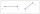Point P is on line segment OQ. Given OP = 6, OQ = 4x - 3, and PQ = 3x, find the numerical length of OQ.
• SixteenSixteen minus the quotient of 12 and what number is thirteen?
• Fraction eq2/3x + 5/7 = 1/2x + 22/21
• A trapezoid 3A trapezoid ABCD has the bases length of a = 120 mm, c = 86 mm and the area A = 2,575 mm2. Find the height of the trapezoid.
• Evaluate expr with 2 varsIf a=6 and x=2, find the value of (2ax + 7x- 10) /(4ax-3a-2)
• The largest divisorThe largest number which divides 60 and 75 leaving remainders 8 and 10 respectively is:
• TrapezoidsIn the isosceles trapezoid ABCD we know: AB||CD, |CD| = c = 8 cm, height h = 7 cm, |∠CAB| = 35°. Find the area of the trapezoid.
• Find a 2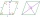Find a length of the diagonal AC of the rhombus ABCD if its perimeter P = 112 dm and the second diagonal BD has a length of 36 dm.
• One of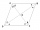One of the internal angles of the rhombus is 120° and the shorter diagonal is 3.4 meters long. Find the perimeter of the rhombus.
• A perimeter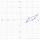A perimeter of a parallelogram is 2.8 meters. The length of one of its sides is equal to one-seventh of the entire perimeter. Find lengths of the sides of the parallelogram.
• An aircraft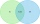An aircraft manufacturing company has submitted bids on two separate airline contracts, A and B. The company feels that it has a 70% chance of winning contract A and a 25% chance of winning contract B. Furthermore, it believes that winning contract A is i
• The ratio 4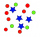The ratio of two number is 5:4 if 40% of the first number is 12, what will be 50% of the second number?
• Perimeter to area 2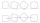Find the area of a square whose perimeter is 100 cm with solve.
• If x=4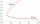If x=4 and y=-3, what is the value of the expression x² + 2xy - 4x + 3y + y²?
• Length 9Length of a rectangle is 8 m less than twice of breadth. If the perimeter of the rectangle is 50. find its length and breadth.
• Eq2 equationsFor each of the following problems, determine the roots of the equation. Given the roots, sketch the graph and explain how your sketch matches the roots given and the form of the equation: g(x)=36x2-12x+5 h(x)=x2-4x+20 f(x)=4x2-24x+45 p(x)=9x2-36x+40
• What are 3What are the two digits which when inserted in the blank spaces will make 234 _ _ divisible by 8?
• Four multiplesFour multiples of 6 he writes the following 12  24  56  72, which is correct?
• Cost structure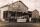You are currently trying to decide between two cost structures for your business: one that has a greater proportion of short-term fixed costs and another that is more heavily weighted to variable costs. Estimated revenue and cost data for each alternative
• Two numbers 11The sum of two rational numbers is (-2). If one of them is 3/5, find the other.

Do you have an exciting math question or word problem that you can't solve? Ask a question or post a math problem, and we can try to solve it.

We will send a solution to your e-mail address. Solved examples are also published here. Please enter the e-mail correctly and check whether you don't have a full mailbox.

Please do not submit problems from current active competitions such as Mathematical Olympiad, correspondence seminars etc...